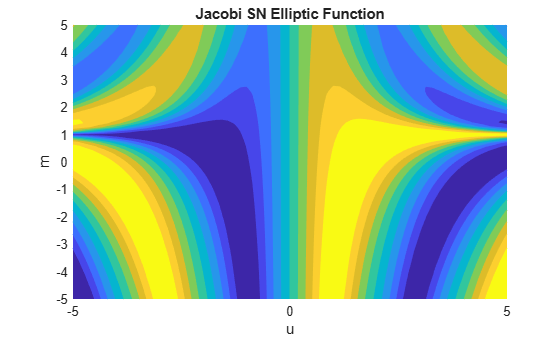# jacobiSN

Jacobi SN elliptic function

## Syntax

``jacobiSN(u,m)``

## Description

example

````jacobiSN(u,m)` returns the Jacobi SN Elliptic Function of `u` and `m`. If `u` or `m` is an array, then `jacobiSN` acts element-wise.```

## Examples

collapse all

`jacobiSN(2,1)`
```ans = 0.9640```

Call `jacobiSN` on array inputs. `jacobiSN` acts element-wise when `u` or `m` is an array.

`jacobiSN([2 1 -3],[1 2 3])`
```ans = 0.9640 0.6721 0.5773```

Convert numeric input to symbolic form using `sym`, and find the Jacobi SN elliptic function. For symbolic input where `u = 0` or `m = 0` or `1`, `jacobiSN` returns exact symbolic output.

`jacobiSN(sym(2),sym(1))`
```ans = tanh(2)```

Show that for other values of `u` or `m`, `jacobiSN` returns an unevaluated function call.

`jacobiSN(sym(2),sym(3))`
```ans = jacobiSN(2, 3)```

For symbolic variables or expressions, `jacobiSN` returns the unevaluated function call.

```syms x y f = jacobiSN(x,y)```
```f = jacobiSN(x, y)```

Substitute values for the variables by using `subs`, and convert values to double by using `double`.

`f = subs(f, [x y], [3 5])`
```f = jacobiSN(3, 5)```
`fVal = double(f)`
```fVal = 0.0311```

Calculate `f` to higher precision using `vpa`.

`fVal = vpa(f)`
```fVal = 0.031144778155397389598324170696454```

Plot the Jacobi SN elliptic function using `fcontour`. Set `u` on the x-axis and `m` on the y-axis by using the symbolic function `f` with the variable order `(u,m)`. Fill plot contours by setting `Fill` to `on`.

```syms f(u,m) f(u,m) = jacobiSN(u,m); fcontour(f,'Fill','on') title('Jacobi SN Elliptic Function') xlabel('u') ylabel('m')```## Input Arguments

collapse all

Input, specified as a number, vector, matrix, or multidimensional array, or a symbolic number, variable, vector, matrix, multidimensional array, function, or expression.

Input, specified as a number, vector, matrix, or multidimensional array, or a symbolic number, variable, vector, matrix, multidimensional array, function, or expression.

collapse all

### Jacobi SN Elliptic Function

The Jacobi SN elliptic function is sn(u,m) = sin(am(u,m)) where am is the Jacobi amplitude function.

The Jacobi elliptic functions are meromorphic and doubly periodic in their first argument with periods 4K(m) and 4iK'(m), where K is the complete elliptic integral of the first kind, implemented as `ellipticK`.

## Version History

Introduced in R2017b